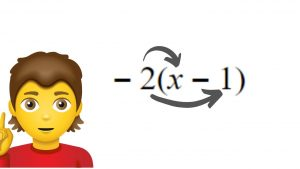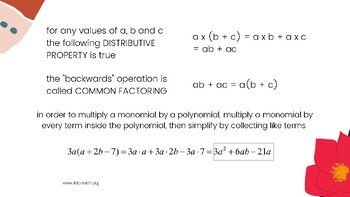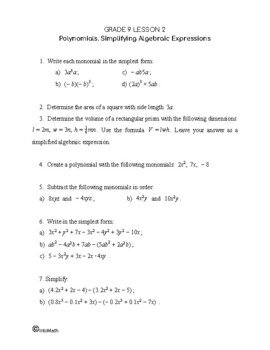Video LessonCA\$2.00

In this lesson we are discovering the distributive property of multiplication, as well as common factoring.

According to the distributive property, a(b + c) = ab + bc.

When multiplying the sum or difference of two terms by another term, it is possible to find the product of each pair of terms first and then add/subtract them.

This is how we expand brackets and simplify algebraic expressions.

​Distributive property is used to simplify expressions, solve equations and word problems. It is a part of virtually every math course in high school and the skill is transferable to other concepts.